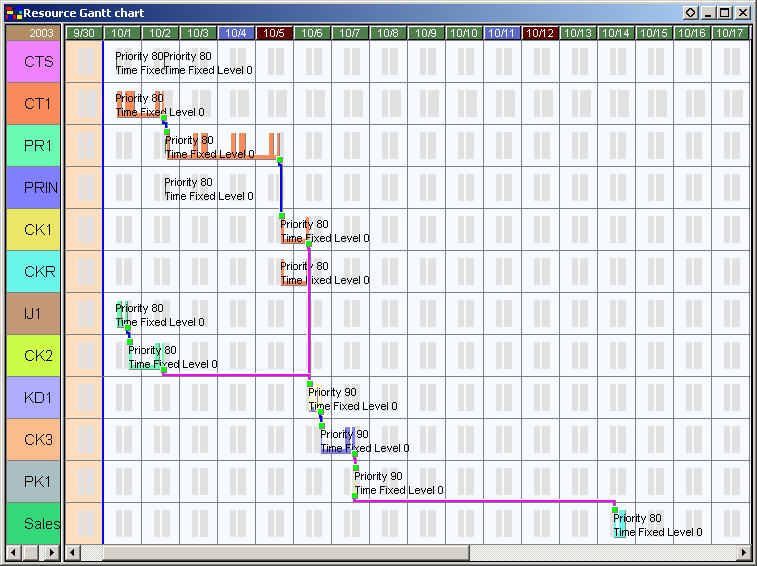## Assignment direction determination and time fixed level

Scheduling Logic

Assign Orders

Assignment Direction

If there are no operations for which results or fixed time levels are specified, assignment direction will be determined according to whether the order is a replenishment order for inventory MIN, as well as the assignment direction of the scheduling parameter, the order and also the order priority.

1. Whether or not the [Assignment direction] property of the Scheduling parameter settings is set to "Forced forward" or "Forced backward"

When the [Assignment direction] property of the Scheduling parameter settings is set to "Forced forward" or "Forced backward", regardless of whether the Time fixed level or not, regardless of whether it is a Safety stock order or not, the assignment will be forcibly made using forward or backward.
(The Forced forward and Forced backward options were added from Ver.6.0.0.)(changed)

2. Replenishment orders for inventory MIN and replenishment orders for target inventory MIN to which no orders are pegged on their right

Orders are assigned forward. If backward assignment is desired, change the properties [Assignment direction of orders for Inventory MIN] / [Assignment direction of orders for Target inventory MIN] in the scheduling parameter to backwards (available from Ver.5.2.0). Other orders will be assigned as below.

3. Scheduling parameters - Assignment direction setting

If "forward" or "backward" is chosen here, then the chosen direction is followed. If "According to order" is chosen, then the direction is decided by one of the methods below.

4. Order table - Assignment direction field setting

If "Forward" or "Backward" is specified here, then the specified direction is followed. If the field is empty, or if "According to priority" is chosen, then the direction is decided by the method below.

5. Order priority field setting

The value of this field determines the direction in the following way:

• Priority < 50 Forward
• 50 <= Priority < 90 Backward
• Priority >= 90 Forward

For example, one order with the priority of 90 is specified as follows: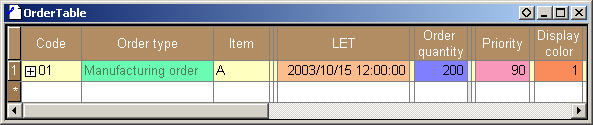If the assignment direction in the scheduling parameter is set to "Unspecified", "According to order", or "Forward", operations will be assigned as follows: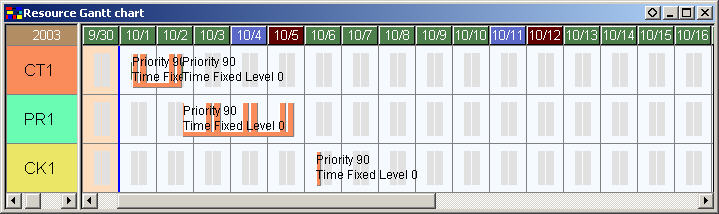If the assignment direction in the scheduling parameter is set to "Backward", then the assignment will result as follows: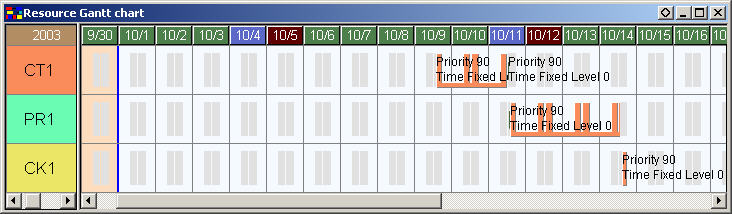Status, the time fixed flag, and assignment direction

Result operations and fixed operations are assigned backward with infinite capacity. Released operations and non-result operations with the time fixed flag are assigned forward with finite capacity.

Time Fixed Level

Each operation has a time fixed level. This time fixed level determines the direction in which the operation is to be assigned.

### Time fixed level determination

 1. If a value is specified for the user specified time fixed level for an operation, this value will be the time fixed level of the operation. 2. Otherwise, the time fixed level depends on the operation's status and other properties as shown in the chart below. 3. Other than the user specified time fixed level, if the time fixed level of an operation overlaps (fixed and frozen, forward first process operation, released, and so on), the larger value will be used.

However, if the following
Forced forward
Forced backward
are set in the [Assignment direction] property of the Scheduling parameter settings, then regardless of the Time fixed level the assignment will be forcibly made using forward or backward.
(The Forced forward and Forced backward options were added from Ver.6.0.0.)

For projects and items, you can specify default values as the fixed level for each of the following status.

 Status, etc. Default Time Fixed Level Completed 50 Started 40 Frozen 30 Released 20 Fixed 10 Scheduled 0

There are two properties for which you can set the time fixed level only to projects.

 Property Name Default Time Fixed Level Forward first process operation 0 Backward final process operation 0

Time Fixed Level and Assignment Direction

The assignment direction is determined based on the operation with the highest time fixed level among the operations of the order. If the operation in the middle process is fixed to the currently assigned time, operations of the previous and next processes will be pulled towards the fixed operation.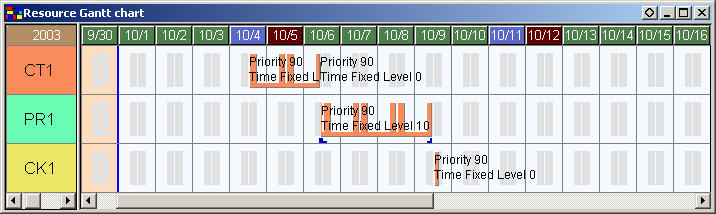If the operations on both ends are fixed with the same time fixed level, and the middle operation appears in between these operations, then the assignment direction of the middle operation will be determined according to the settings of the scheduling parameter and the order. In this example, since Assignment direction of the scheduling parameter = "According to order priority" and Priority = 90, the middle operation will be assigned forward.If Assignment direction of the scheduling parameter = "According to order" and Priority = 80, the assignment direction of the middle operation will be backward.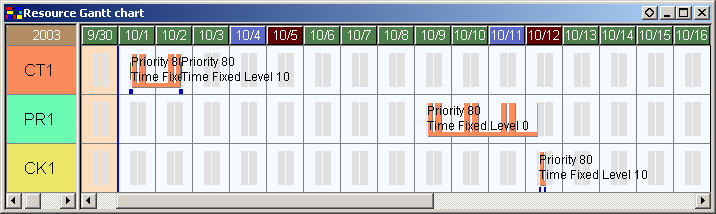If the operations on both ends are fixed with different time fixed levels, then the middle operation between them will be always pulled towards the one with higher fixed level. In this example, since the previous operation is frozen (the time fixed level is 30) and the next operation is fixed (the time fixed level is 10), the middle operation is pulled towards the previous operation.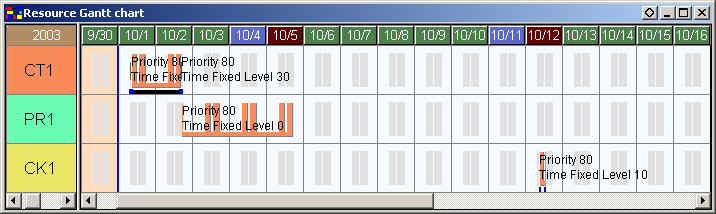In the case where the assignment direction is set without fixing time, if results are entered in the first process, then the operations in the following processes will be assigned forward even if the order is supposed to be assigned backward.If you set [Time fixed level (Backward final process)] in the project settings to "60" (higher time fixed level than that of results), you can assign the operations in the later processes backward.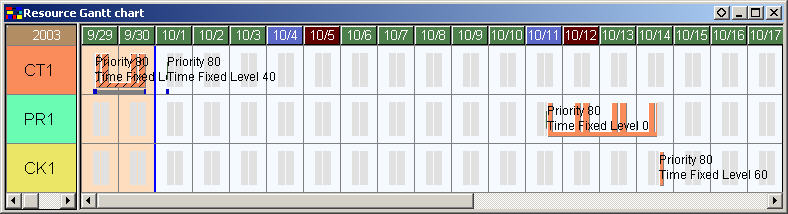If you change [Priority] to 90, the assignment direction will be forward. In that case, "Time fixed level (Backward final process)" is disabled and the operations will be assigned forward.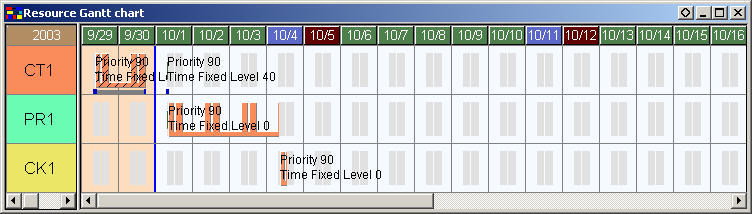Example of Scheduling parameter settings for Assignment direction, "Forced forward" and "Forced backward"

If the next process operations is fixed, then the assignment would normally be backward. Since the Scheduling parameter setting "Assignment direction" has been set to "Forced forward", the assignment will start from the order's EST with Forced forward assignment.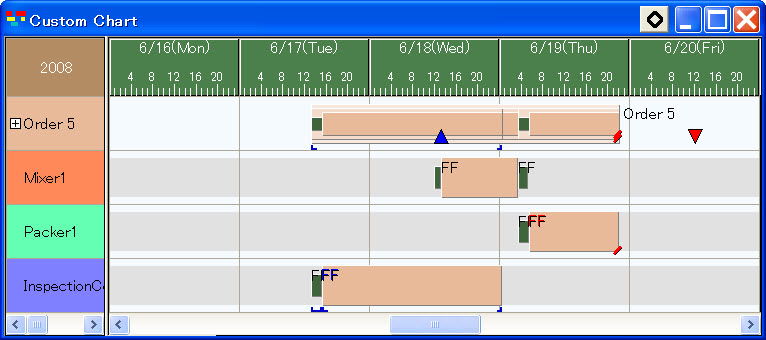If the previous process operations is fixed, then the assignment would normally be forward. Since the Scheduling parameter setting "Assignment direction" has been set to "Forced backward", the assignment will start from the order's due date with Forced backward assignment.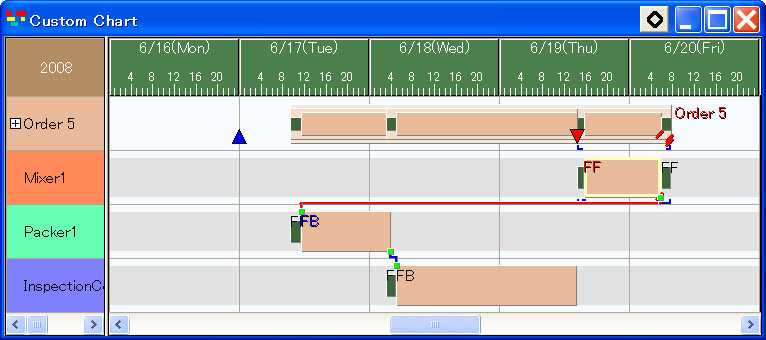Assignment direction when multiple orders are pegged (according to item or next order constraints)

Basically, the assignment direction is determined according to the time fixed level of operations within the order.

For example, orders are set as follows: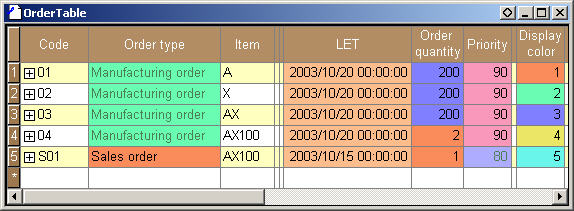When the time fixed level of all operations is "0"

The assignment direction of the operations will be determined according to the scheduling parameter, order priority and assignment direction of the order.

In the above order settings, if the assignment direction of the scheduling parameter is set to "Unspecified", "According to order priority", or "Forward", then the assignment of the operations will result as follows: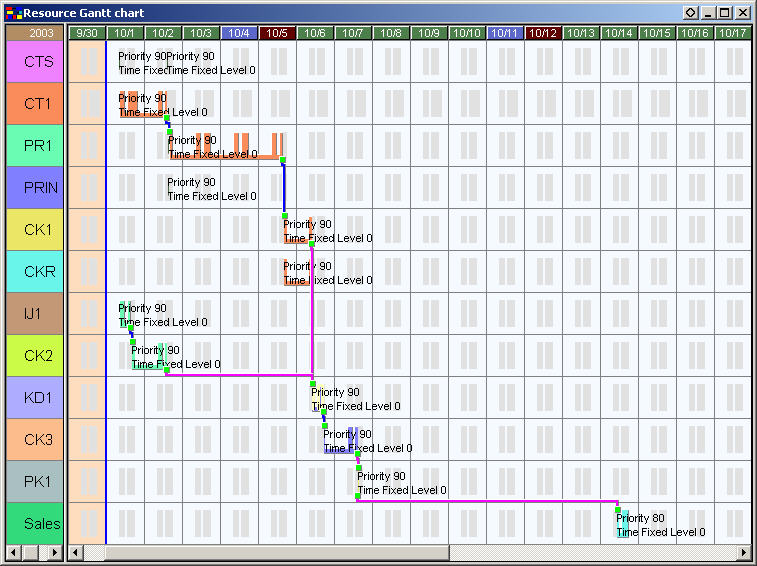If the assignment direction of the scheduling parameter is changed to "Backward", then the result of rescheduling will look as follows: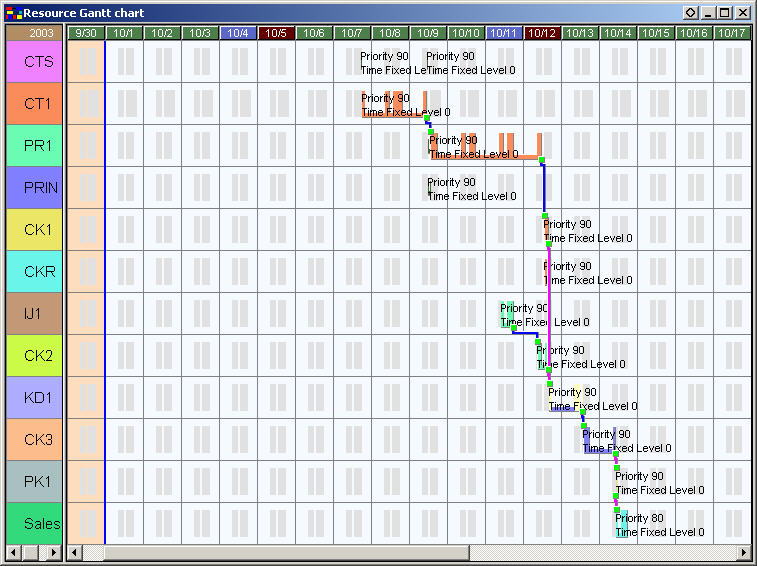To assign orders for the earlier processes as forward and the later processes to "Backward", we will change the order priorities as follows.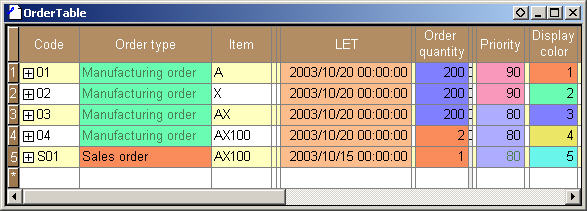If the assignment direction of the scheduling parameter is set to "Unspecified" or "According to order priority", then the operations will be assigned as follows: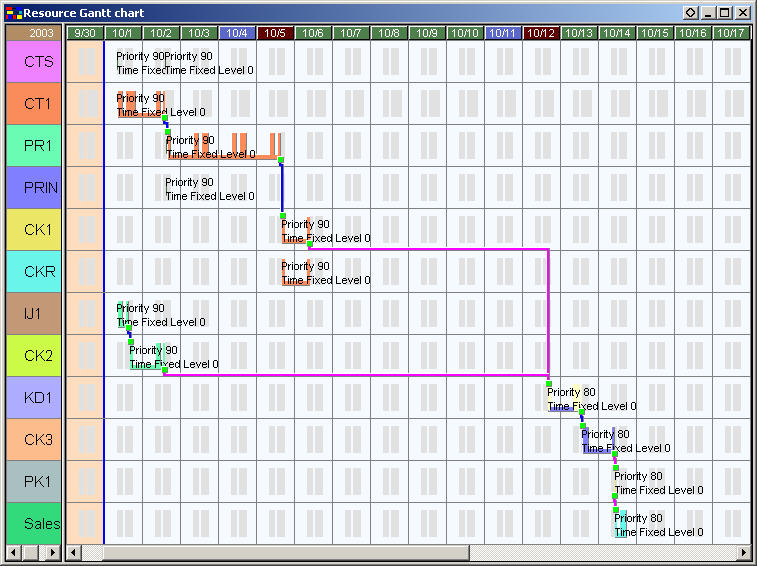If we move an operation in earlier process as below and then to reschedule, the operations in the later processes will be assigned "Backward" and an inconsistent time relationship between the processes will occur.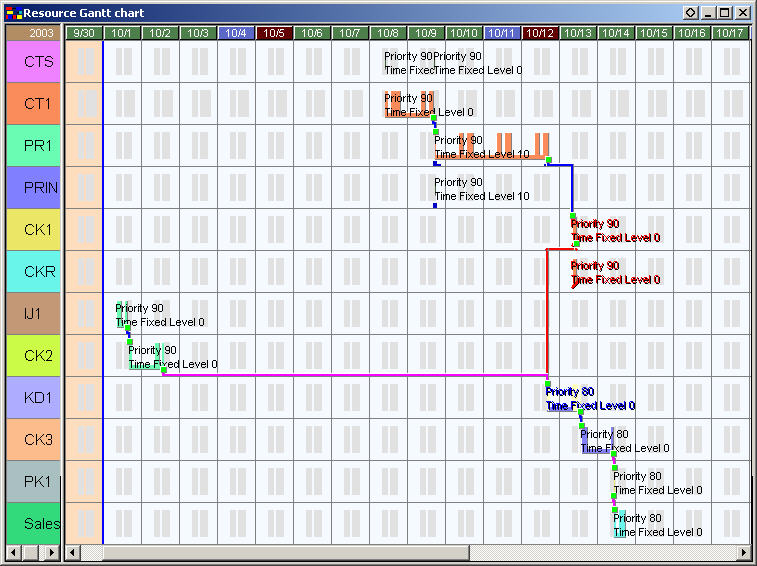If you set "Passed EST" in the scheduling parameter to "Switch to forward" and then to reschedule, the adjustment command will switch the direction and the above inconsistency will disappear.Now, change the order priorities as follows to assign orders for earlier processes to "Backward" and later processes to "Forward".For backward operations, the assignment direction is changed to "Forward". The assignment direction is determined proceeding from the operation with the lowest LLC and other operations may sometimes be assigned depending on the assignment direction of those assigned before the operations. In the following sample, the assignment direction of orders "04" and "03" is determined first and the assignment direction of orders "01" and "02" is determined later. However, if "Forward" operations exist to the right of orders "01" and "02", the orders cannot be assigned "Backward" and the assignment direction of the orders will be "Forced forward".